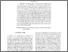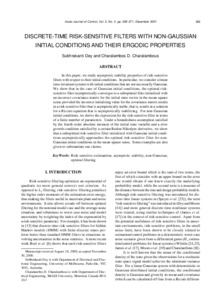# Discrete-time risk-sensitive filters with non-gaussian initial conditions and their Ergodic properties.

Dey, Subhrakanti and Charalambous, Charalambos D. (2001) Discrete-time risk-sensitive filters with non-gaussian initial conditions and their Ergodic properties. Asian Journal of Control, 3 (4). pp. 262-271. ISSN 1563-8625Previewmore...Add this article to your Mendeley library

## Abstract

In this paper, we study asymptotic stability properties of risk-sensitive filters with respect to their initial conditions. In particular, we consider a linear time-invariant systems with initial conditions that are not necessarily Gaussian. We show that in the case of Gaussian initial conditions, the optimal risksensitive filter asymptotically converges to a suboptimal filter initialized with an incorrect covariance matrix for the initial state vector in the mean square sense provided the incorrect initializing value for the covariance matrix results in a risk-sensitive filter that is asymptotically stable, that is, results in a solution for a Riccati equation that is asymptotically stabilizing. For non-Gaussian initial conditions, we derive the expression for the risk-sensitive filter in terms of a finite number of parameters. Under a boundedness assumption satisfied by the fourth order absolute moment of the initial state variable and a slow growth condition satisfied by a certain Radon-Nikodym derivative, we show that a suboptimal risk-sensitive filter initialized with Gaussian initial conditions asymptotically approaches the optimal risk-sensitive filter for nonGaussian initial conditions in the mean square sense. Some examples are also given to substantiate our claims.

Item Type: Article Risk-sensitive estimantion; asymptotic stability; non-Gaussian; optimal filtering; Faculty of Science and Engineering > Electronic Engineering 12731 Subhrakanti Dey 09 Apr 2020 10:13 Asian Journal of Control Wiley YesItem control page